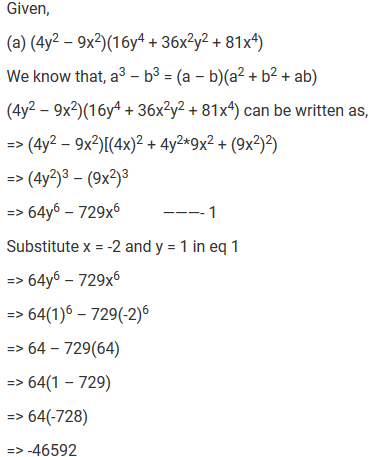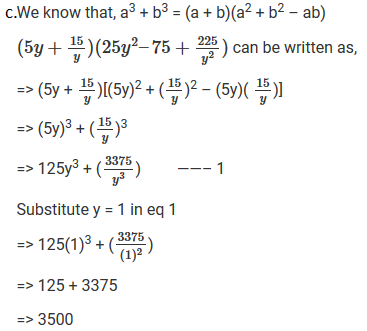#### Chapter 4 Algebraic Identities R.D. Sharma Solutions for Class 9th Math Exercise 4.4

Exercise 4.4

1. Find the following products:Solution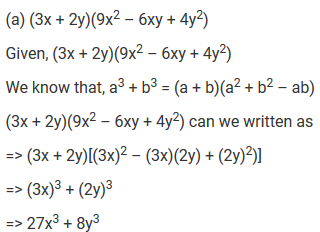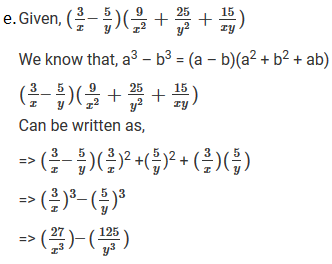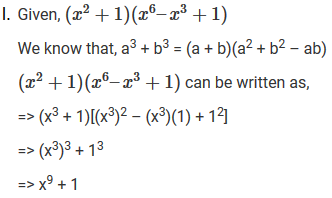2. If x = 3 and y = − 1, find the values of each of the following using in identify:Solution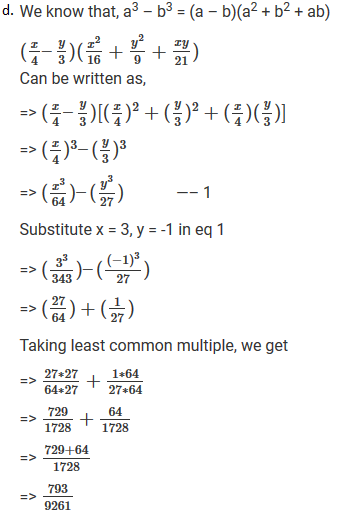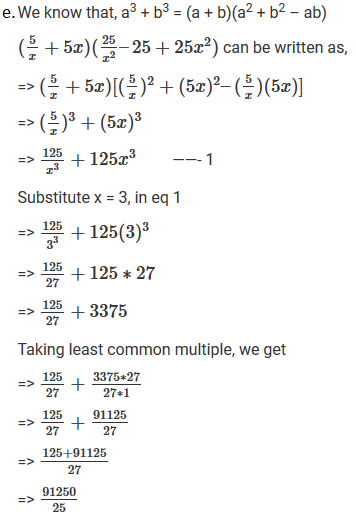3. If a + b = 10 and ab = 16, find the value of a2 − ab + b2 and a2 + ab + b2

Solution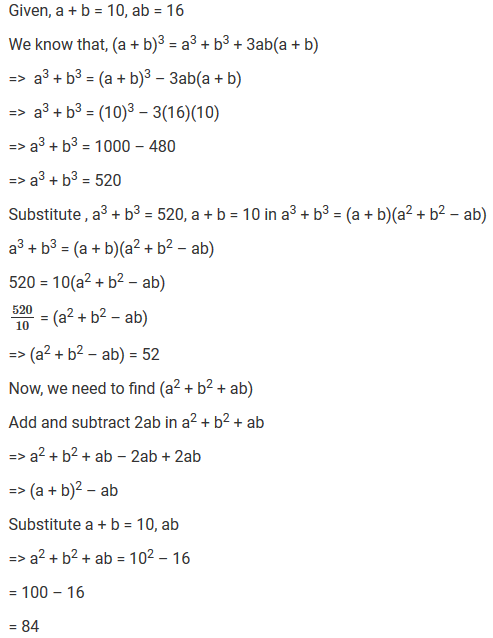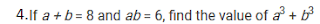SolutionSolution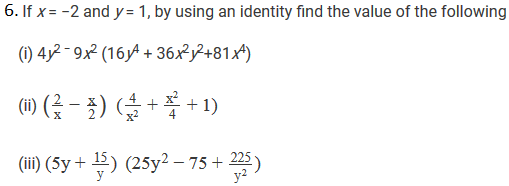Solution